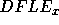Next: Disability prevalences for Sullivan Up: Annex: Variance of Sullivan Previous: Estimate of variance of

## Example of variance calculation

Here, we are going to give an example of calculation of the variance of Sullivan index. We use equation 21 on French data (life tables of 1991, males, prevalence ratios derived from health survey).

However, in order to show the use of some previous equations, we have complicated the example by assuming that the survey didn't take into account institutionalized persons. If we use the prevalence ratios directly derived from the survey in the formula 1, we make the implicit assumption that the prevalence ratios in institutionalized population are the same as in the non institutionalized population. Because, we do not want to do such an assumption in the calculation of, we are going to estimate the variance of the disability free and non institutionalized life expectancy. To that extent, it is assumed that formula 8 has been used to calculatein section 1.3.

Eric Hauet
Fri Apr 25 22:40:35 DFT 1997